# 机器学习# 一、学习目标

1. 了解人工智能、机器学习和深度学习之间的区别
2. 掌握机器学习中的监督学习和无监督学习问题

# 二、人工智能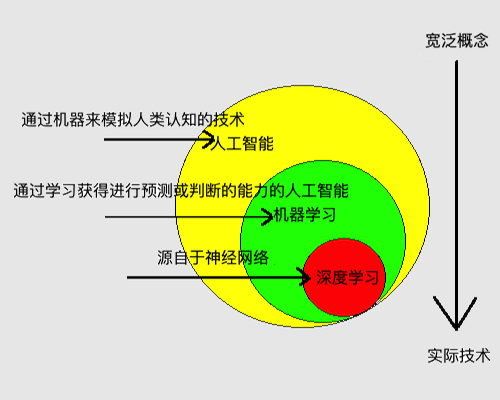1. 人脸识别应用中，他根据输入的照片，判断照片中的人是谁
2. 医疗诊断中，他根据输入的医疗影像，判断疾病的成因
3. 语音识别中，他根据人说话的音频信号，判断说话的内容
4. 电子商务网站中，他根据用户曾经的购买记录，预测用户感兴趣的商品，网站进而达到推荐商品的目的
5. 自动驾驶应用中，他根据对当前汽车所处情况的分析，判断汽车接下来的速度和方向
6. 金融应用中，他根据股票曾经的价格和其他交易信息，预测股票未来的价格走势
7. 围棋对弈中，他根据当前的盘面形式，预测在哪个地方落子胜率最大（2016年3月的alphago大战李世石）
8. 基因测序应用中，他根据对人的基因序列的分析，预测这个人未来患病的可能性
9. 智能家居、智能玩具、网络安全……

# 三、机器学习

## 3.1 机器学习基本术语

# 机器学习基本术语图例
import matplotlib.pyplot as plt
from matplotlib.font_manager import FontProperties
# 显示plt.show()的图片，如若不使用jupyter，请注释
%matplotlib inline
# 中文字体设置，找到与之对应的中文字体路径
font = FontProperties(fname='/Library/Fonts/Heiti.ttc')

# 样本
plt.text(1, 70, s='{身高(180)，体重(70)，年龄(19)，五官(精致)',
fontproperties=font, fontsize=13, color='g')
plt.annotate(text='样本', xytext=(90, 70), xy=(78, 71), ha='center', arrowprops=dict(
arrowstyle="<-", color='b'), fontproperties=font, fontsize=15, color='r')
plt.annotate(text='特征', xytext=(30, 90), xy=(10, 73), ha='center', arrowprops=dict(
arrowstyle="<-", color='b'), fontproperties=font, fontsize=15, color='r')
plt.annotate(text='', xytext=(30, 90), xy=(30, 73), ha='center', arrowprops=dict(
arrowstyle="<-", color='b'), fontproperties=font, fontsize=15, color='r')
plt.annotate(text='', xytext=(30, 90), xy=(50, 73), ha='center', arrowprops=dict(
arrowstyle="<-", color='b'), fontproperties=font, fontsize=15, color='r')
plt.annotate(text='', xytext=(30, 90), xy=(70, 73), ha='center', arrowprops=dict(
arrowstyle="<-", color='b'), fontproperties=font, fontsize=15, color='r')

plt.hlines(67, 0, 100, linestyle='--', color='gray')

# 特征
plt.text(1, 40, s='{身高(180)，体重(70)，年龄(19)，五官(精致)，帅}',
fontproperties=font, fontsize=10, color='g')
plt.annotate(text='样例(实例)', xytext=(90, 40), xy=(70, 41), ha='center', arrowprops=dict(
arrowstyle="<-", color='b'), fontproperties=font, fontsize=15, color='r')
plt.annotate(text='特征', xytext=(30, 60), xy=(8, 43), ha='center', arrowprops=dict(
arrowstyle="<-", color='b'), fontproperties=font, fontsize=15, color='r')
plt.annotate(text='', xytext=(30, 60), xy=(23, 43), ha='center', arrowprops=dict(
arrowstyle="<-", color='b'), fontproperties=font, fontsize=15, color='r')
plt.annotate(text='', xytext=(30, 60), xy=(38, 43), ha='center', arrowprops=dict(
arrowstyle="<-", color='b'), fontproperties=font, fontsize=15, color='r')
plt.annotate(text='', xytext=(30, 60), xy=(53, 43), ha='center', arrowprops=dict(
arrowstyle="<-", color='b'), fontproperties=font, fontsize=15, color='r')
plt.annotate(text='标记', xytext=(67, 60), xy=(67, 43), ha='center', arrowprops=dict(
arrowstyle="<-", color='b'), fontproperties=font, fontsize=15, color='r')

plt.hlines(37, 0, 100, linestyle='--', color='gray')

# 特征空间
plt.text(1, 30, s='$\{(x_1^{(1)},x_1^{(2)},\cdots,x_1^{(n)}),(x_2^{(1)},x_2^{(2)},\cdots,x_2^{(n)}),\cdots,(x_m^{(1)},x_m^{(2)},\cdots,x_m^{(n)})\}$',
fontproperties=font, fontsize=10, color='g')
plt.annotate(text='特征空间($m$个样本)', xytext=(90, 15), xy=(92, 30), ha='center', arrowprops=dict(
arrowstyle="<-", color='b'), fontproperties=font, fontsize=15, color='r')
plt.annotate(text='特征向量($n$维特征)', xytext=(15, 15), xy=(15, 28), ha='center', arrowprops=dict(
arrowstyle="<-", color='b'), fontproperties=font, fontsize=15, color='r')

plt.xlim(0, 100)
plt.ylim(10, 100)
plt.title('机器学习基本术语图例', fontproperties=font, fontsize=20)
plt.show()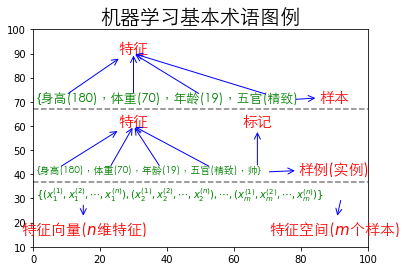• 特征（feature）：描述一件事物的特性，如一个人的身高、体重、年龄和五官。
• 样本（sample）：由一个人的特征组成的数据，如$$\{180,70,19,精致\}$$
• 标记（label）：描述一件事物的特性，如一个人帅或丑、一个人的财富数量。注：特征和标记没有明确的划分，由于问题的不同可能导致A问题的特征是B问题的标记，B问题的标记是A问题的特征。
• 样例（example）：由一个人的特征和标记组成的数据，如$$\{180,70,19,精致,帅\}$$
• 特征空间（feature space）：由$$\{x^{(1)},x^{(2)},\cdots,x^{(n)}\}$$这n个特征张成的n维空间，如以身高张成的一维空间（线）；以身高和体重张成的二维空间（面）；以身高、体重和年龄张成的三维空间（体）。
• 特征向量（feature vector）：特征空间内的某一个具体的向量，即特种空间中的某一个具体的点，

$\{x_i^{(1)},x_i^{(2)},\cdots,x_i^{(n)}\},\,i\in{R}$

# 四、深度学习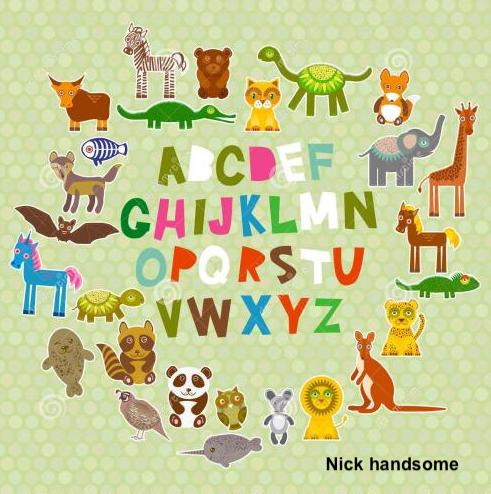# 五、机器学习分类## 5.1 监督学习

1. 输入：一组已知类别的样本
2. 输出：一个模型
3. 预测未来新数据的预测值或预测类别

• 如果模型的输出为连续值，如股票价格$$2.2,2.4,2.6,3…$$类型的数据称为连续值，则该监督学习问题称作回归问题
• 如果模型的输出为离散值，如$$阿猫,阿狗,…$$$$\{0,1\}$$（输出不是$$0$$就是$$1$$），则该监督学习问题称作分类问题。

### 5.1.1 回归问题

1 10 100
2 20 200
3 40 400
4 100 1000
5 200 2000
# 回归问题图例
import numpy as np
import matplotlib.pyplot as plt
from matplotlib.font_manager import FontProperties
%matplotlib inline
font = FontProperties(fname='/Library/Fonts/Heiti.ttc')

x = np.linspace(0, 200, 10)
y = 10*x

plt.plot(x, y)
plt.xlabel('房子面积', fontproperties=font, fontsize=15)
plt.ylabel('房价', fontproperties=font, fontsize=15)
plt.title('回归问题图例', fontproperties=font, fontsize=20)
plt.show()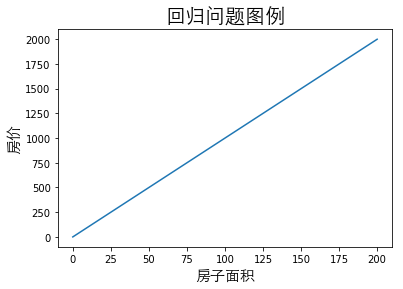$房价=10*房子面积$

$H_1的房价=10*H_1的房子面积=10*1000=10000(元)\quad\text{预测值}$

1. 房子面积称为特征（feature）
2. 房价称为标记（label）

### 5.1.2 分类问题

1 10 A区
2 20 A区
3 40 A区
4 100 B区
5 200 B区
# 分类问题图例
import numpy as np
import matplotlib.pyplot as plt
from matplotlib.font_manager import FontProperties
%matplotlib inline
font = FontProperties(fname='/Library/Fonts/Heiti.ttc')

a_area = [10, 20, 40]
a_y = [0, 0, 0]

b_area = [100, 200]
b_y = [0, 0]

plt.scatter(a_area, a_y, label='A', color='r')
plt.scatter(b_area, b_y, label='B', color='g')
plt.vlines(50, ymin=-0.015, ymax=0.015, color='gray', linestyles='--')
plt.xlabel('房子面积', fontproperties=font, fontsize=15)
plt.title('分类问题图例', fontproperties=font, fontsize=20)
plt.legend()
plt.show()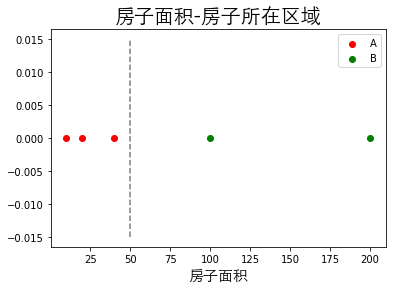$房子所在区域 = \begin{cases} P(A区|房子面积<50m^2) \\ P(B区|房子面积>50m^2) \end{cases}$

$\begin{cases} 因为H_1的面积\,<50m^2,&H_1属于A区的概率为1,H_1属于B区的概率为0\quad\text{预测类别} \\ 因为H_2的面积\,>50m^2,&H_2属于B区的概率为1,H_2属于A区的概率为0\quad\text{预测类别} \end{cases}$

1. 房子面积称为特征
2. 房子所在区域称为标记

## 5.2 无监督学习

1. 输入：一组未知类别的样本
2. 输出：分成多个簇的一组样本

1 10
2 20
3 40
4 100
5 200

## 5.3 半监督学习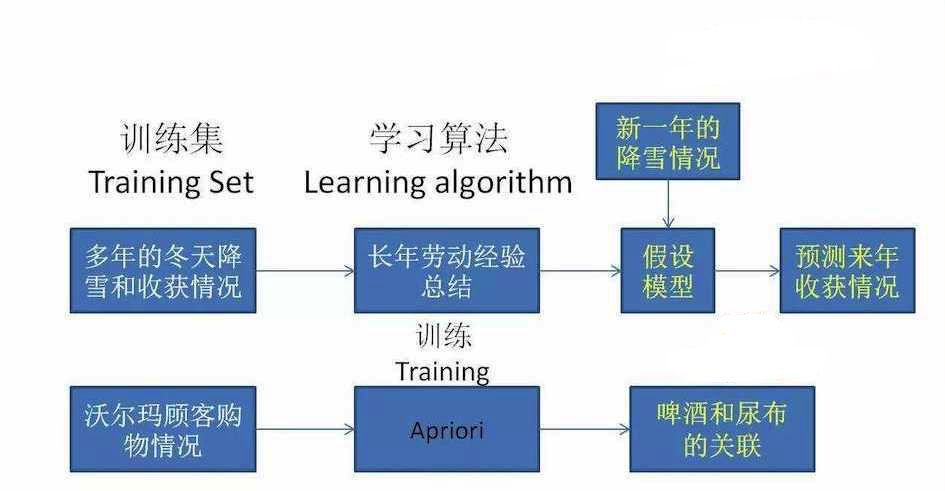# 六、Python语言的优势

• numpy 1.15.2
• scipy 1.1.0
• scikit-learn 0.20.0
• matplotlib 3.0.2
• xlrd 1.2.0
• pandas 0.23.4
• tensorflow 1.1.0
# 终端输入，安装numpy，其他第三库同理
!pip install numpy==1.15.2

Requirement already satisfied: numpy==1.15.2 in /Applications/anaconda3/lib/python3.6/site-packages (1.15.2)

import numpy

# 打印sklearn的版本
numpy.__version__

'1.15.2'


# 七、小结

posted @ 2019-10-16 17:07  B站-水论文的程序猿  阅读(11316)  评论(0编辑  收藏  举报